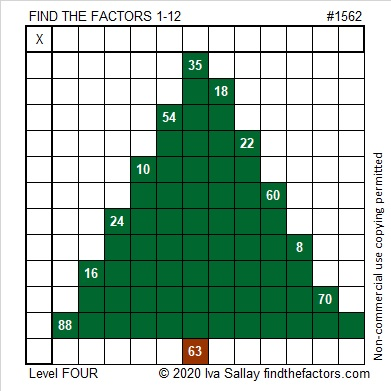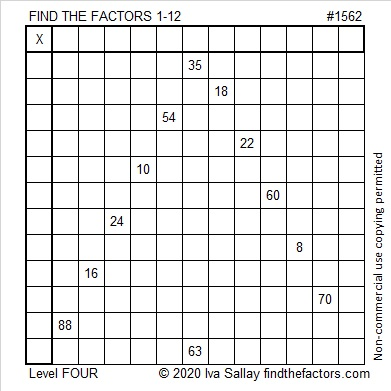# 1562 Evergreen Tree

Contents

### Today’s Puzzle:

An evergreen tree doesn’t drop its leaves in the fall or look dead in the winter. As it reminds us of everlasting life, it makes a lovely symbol of Christmas.Write the numbers from 1 to 10 in both the first column and the top row so that those numbers and the given clues function like a multiplication table. Here is the same puzzle that won’t use as much ink to print:### Factors of 1562:

• 1562 is a composite number.
• Prime factorization: 1562 = 2 × 11 × 71.
• 1562 has no exponents greater than 1 in its prime factorization, so √1562 cannot be simplified.
• The exponents in the prime factorization are 1, 1, and 1. Adding one to each exponent and multiplying we get (1 + 1)(1 + 1)(1 + 1) = 2 × 2 × 2 = 8. Therefore 1562 has exactly 8 factors.
• The factors of 1562 are outlined with their factor pair partners in the graphic below.### Another Fact about the Number 1562:

2(5⁴ + 5³ + 5² + 5¹ + 5⁰) = 1562

This site uses Akismet to reduce spam. Learn how your comment data is processed.